Command Reference : Operator and Function Reference : Moving Statistic Functions

Moving Statistic Functions
These functions perform basic rolling or moving statistics. They may be used as part of a series expression.
The moving statistic functions are in two types, those that propagate missing observations (NAs) and those that don’t. The functions that do not propagate NAs, which start with “@m”, skip observations which are NA. The functions that do propagate NAs will return NA when an NA is encountered.
For example if the series X contains {1, 3, 4, NA, 5, 3, 2} then “@movav(x,2)” will give {NA, 2, 3.5, NA, NA, 4, 2.5}, whereas “@mav(x,2)” will give {1, 2, 3.5, 4, 5, 4, 2.5}.

 Name Function Description @movsum(x,n) n-period backward moving sum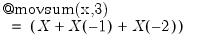. NAs are propagated. @movav(x,n) n-period backward moving average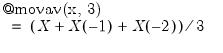NAs are propagated. @movavc(x,n) n-period centered moving average centered moving average of X. Note if n is even then the window length is increased by one and the two endpoints are weighted by 0.5. NAs are propagated. @movstdev(x,n) n-period backwards moving standard deviation sample standard deviation (division by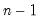) of X for the current and previousobservations. NAs are propagated. @movstdevs(x,n) n-period backwards moving sample standard deviation sample standard deviation (division by) of X for the current and previousobservations. Note this is the same calculation as @movstdev. NAs are propagated. @movstdevp(x,n) n-period backwards moving population standard deviation population standard deviation (division by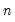) of X for the current and previousobservations. NAs are propagated. @movvar(x,n) n-period backwards moving variance population variance (division by) of X for the for the current and previousobservations. NAs are propagated. @movvars(x,n) n-period backwards moving sample variance sample variance (division by) of X for the current and previousobservations. NAs are propagated. @movvarp(x,n) n-period backwards moving population variance population variance (division by).of X for the current and previousobservations. Note this is the same calculation as @movvar. NAs are propagated. @movcov(x,y,n) n-period backwards moving covariance population covariance (division by) between X and Y of the current and previousobservations. NAs are propagated. @movcovs(x,y,n) n-period backwards moving sample covariance sample covariance (division by) between X and Y of the current and previousobservations. NAs are propagated. @movcovp(x,y,n) n-period backwards moving population covariance population covariance (division by) between X and Y of the current and previousobservations. Note this is the same calculation as @movcov. NAs are propagated. @movcor(x,y,n) n-period backwards moving correlation correlation between X and Y of the current and previousobservations. NAs are propagated. NAs are propagated. @movmax(x,n) n-period backwards moving maximum the maximum of X for the current and previousobservations. NAs are propagated. @movmin(x,n) n-period backwards moving minimum the minimum of X for the current and previousobservations. NAs are propagated. @movsumsq(x,n) n-period backwards sum-of-squares the sum-of-squares of X for the current and previousobservations. NAs are propagated. @movskew(x,n) n-period backwards skewness the skewness of X for the current and previousobservations. NAs are propagated. @movkurt(x,n) n-period backwards kurtosis the kurtosis of X for the current and previousobservations. NAs are propagated. @movobs(x,n) n-period backwards nmber of non-NA observations the number of non-missing observations in X for the current and previousobservations. Note this function always returns the same value as @mobs. @movnas(x,n) n-period backwards nmber of NA observations the number of missing observations in X for the current and previous n-1 observations. Note this function always returns the same value as @mnas. @movinner(x,y,n) n-period backwards inner product of X and Y inner product of X and Y for the current and previousobservations. NAs are propagated. @msum(x,n) n-period backward moving sum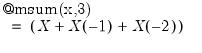NAs are not propagated. @mav(x,n) n-period backward moving average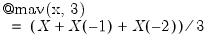NAs are not propagated. @mavc(x,n) n-period centered moving average centered moving average of X. Note if n is even then the window length is increased by one and the two endpoints are weighted by 0.5. NAs are not propagated. @mstdev(x,n) n-period backwards moving standard deviation sample standard deviation (division by) of X for the current and previousobservations. NAs are not propagated. @mstdevs(x,n) n-period backwards moving sample standard deviation sample standard deviation (division by) of X for the current and previousobservations. Note this is the same calculation as @movstdev. NAs are not propagated. @mstdevp(x,n) n-period backwards moving population standard deviation population standard deviation (division by) of X for the current and previousobservations. NAs are not propagated. @mvar(x,n) n-period backwards moving variance population variance (division by) of X for the for the current and previousobservations. NAs are not propagated. @mvars(x,n) n-period backwards moving sample variance sample variance (division by) of X for the current and previousobservations. NAs are not propagated. @mvarp(x,n) n-period backwards moving population variance population variance (division by) of X for the current and previousobservations. Note this is the same calculation as @movvar. NAs are not propagated. @mcov(x,y,n) n-period backwards moving covariance population covariance (division by) between X and Y of the current and previousobservations. NAs are not propagated. @mcovs(x,y,n) n-period backwards moving sample covariance sample covariance (division by) between X and Y of the current and previousobservations. NAs are not propagated. @mcovp(x,y,n) n-period backwards moving population covariance population covariance (division by) between X and Y of the current and previousobservations. Note this is the same calculation as @movcov. NAs are not propagated. @mcor(x,y,n) n-period backwards moving correlation correlation between X and Y of the current and previousobservations. NAs are not propagated. @mmax(x,n) n-period backwards moving maximum the maximum of X for the current and previousobservations. NAs are not propagated. @mmedian(x,n) n-period backward moving median the median of X for the current and previousobservations. NAs are not propagated. @mmin(x,n) n-period backwards moving minimum the minimum of X for the current and previousobservations. NAs are not propagated. @msumsq(x,n) n-period backwards sum-of-squares the sum-of-squares of X for the current and previousobservations. NAs are not propagated. @mskew(x,n) n-period backwards skewness the skewness of X for the current and previousobservations. NAs are not propagated. @mkurt(x,n) n-period backwards kurtosis the kurtosis of X for the current and previousobservations. NAs are not propagated. @mobs(x,n) n-period backwards number of non-NA observations the number of non-missing observations in X for the current and previousobservations. Note this function always returns the same value as @movobs. @mnas(x,n) n-period backwards number of NA observations the number of missing observations in X for the current and previousobservations. Note this function always returns the same value as @movnas. @minner(x,y,n) n-period backwards inner product of X and Y inner product of X and Y for the current and previousobservations. NAs are not propagated.